Скачать презентацию LECTURE 16 Class Cohesion Metrics Ivan Marsic Rutgers

e570e13a7bee9320d3b692789ed936e2.ppt

• Количество слайдов: 22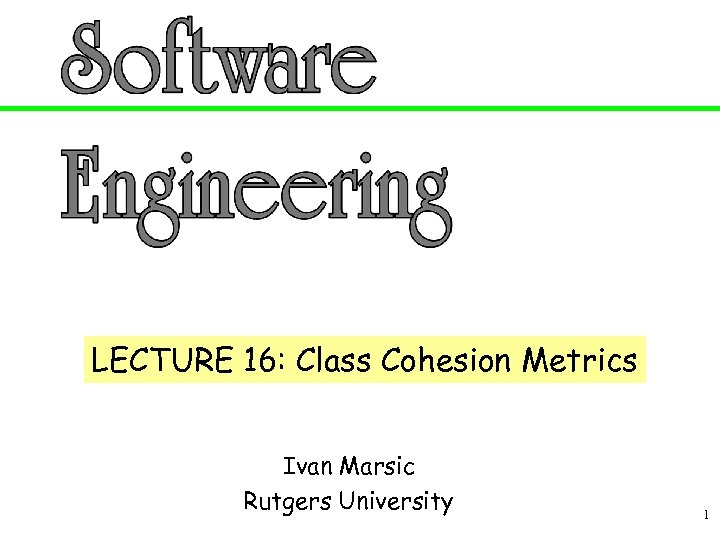LECTURE 16: Class Cohesion Metrics Ivan Marsic Rutgers University 1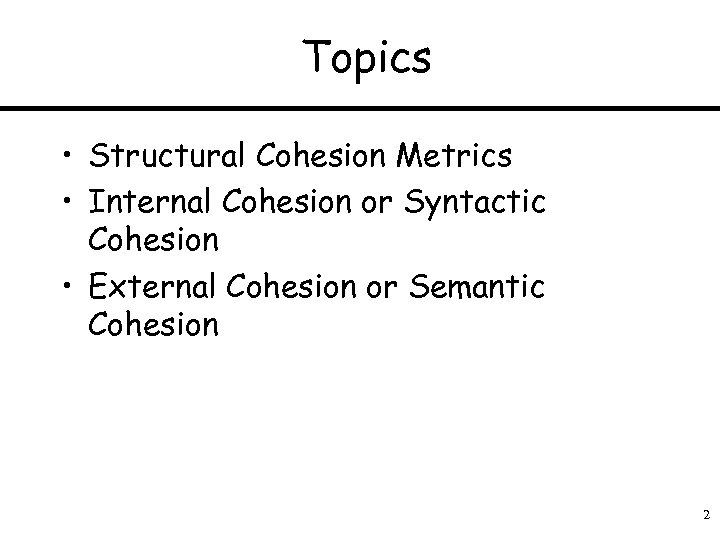Topics • Structural Cohesion Metrics • Internal Cohesion or Syntactic Cohesion • External Cohesion or Semantic Cohesion 2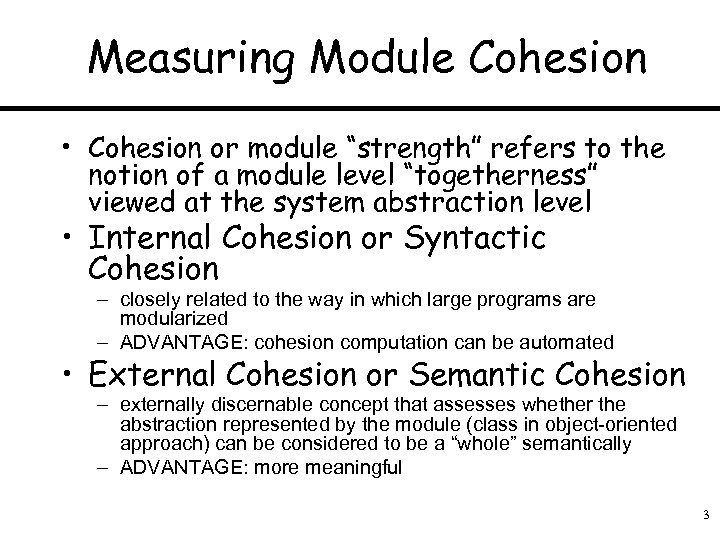Measuring Module Cohesion • Cohesion or module “strength” refers to the notion of a module level “togetherness” viewed at the system abstraction level • Internal Cohesion or Syntactic Cohesion – closely related to the way in which large programs are modularized – ADVANTAGE: cohesion computation can be automated • External Cohesion or Semantic Cohesion – externally discernable concept that assesses whether the abstraction represented by the module (class in object-oriented approach) can be considered to be a “whole” semantically – ADVANTAGE: more meaningful 3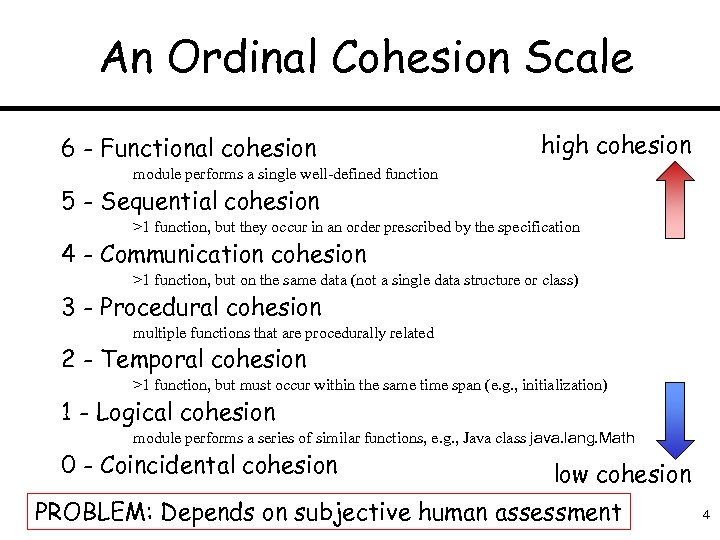An Ordinal Cohesion Scale 6 - Functional cohesion high cohesion module performs a single well-defined function 5 - Sequential cohesion >1 function, but they occur in an order prescribed by the specification 4 - Communication cohesion >1 function, but on the same data (not a single data structure or class) 3 - Procedural cohesion multiple functions that are procedurally related 2 - Temporal cohesion >1 function, but must occur within the same time span (e. g. , initialization) 1 - Logical cohesion module performs a series of similar functions, e. g. , Java class java. lang. Math 0 - Coincidental cohesion low cohesion PROBLEM: Depends on subjective human assessment 4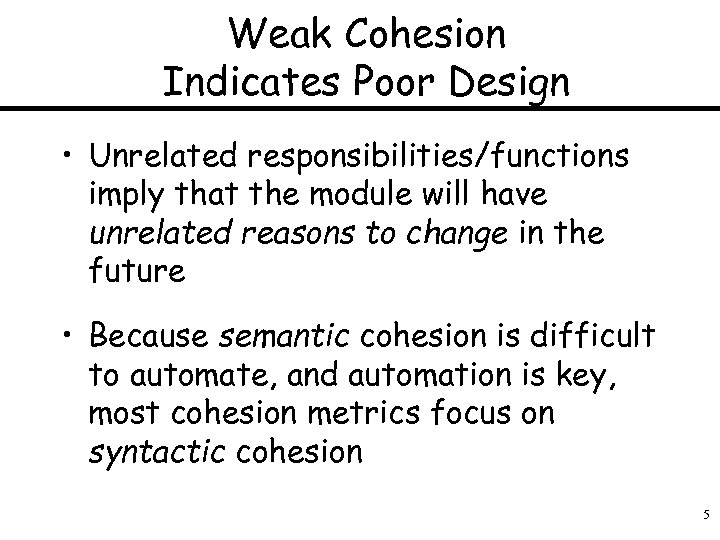Weak Cohesion Indicates Poor Design • Unrelated responsibilities/functions imply that the module will have unrelated reasons to change in the future • Because semantic cohesion is difficult to automate, and automation is key, most cohesion metrics focus on syntactic cohesion 5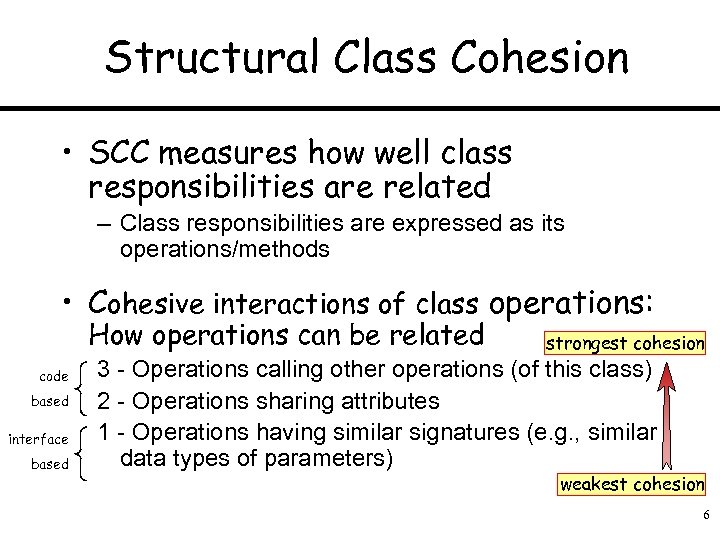Structural Class Cohesion • SCC measures how well class responsibilities are related – Class responsibilities are expressed as its operations/methods • Cohesive interactions of class operations: How operations can be related code based interface based strongest cohesion 3 - Operations calling other operations (of this class) 2 - Operations sharing attributes 1 - Operations having similar signatures (e. g. , similar data types of parameters) weakest cohesion 6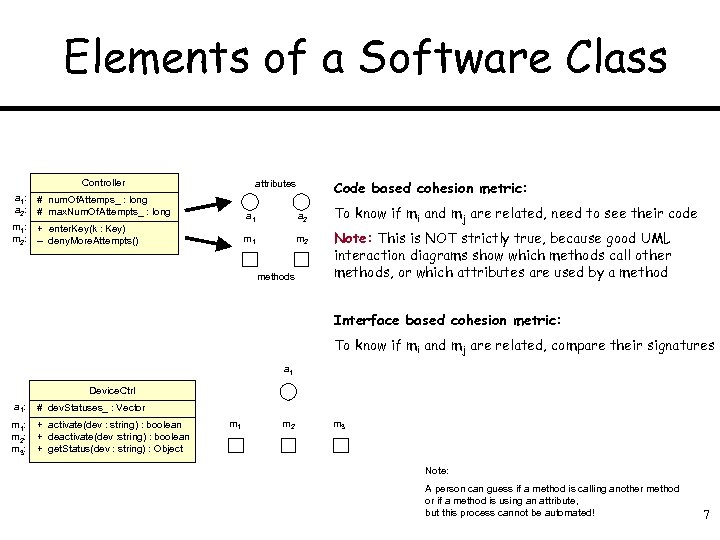Elements of a Software Class Controller a 1: a 2: # num. Of. Attemps_ : long # max. Num. Of. Attempts_ : long m 1: m 2: Code based cohesion metric: attributes + enter. Key(k : Key) – deny. More. Attempts() a 1 a 2 m 1 m 2 methods To know if mi and mj are related, need to see their code Note: This is NOT strictly true, because good UML interaction diagrams show which methods call other methods, or which attributes are used by a method Interface based cohesion metric: To know if mi and mj are related, compare their signatures a 1 Device. Ctrl a 1: # dev. Statuses_ : Vector m 1: m 2: m 3: + activate(dev : string) : boolean + deactivate(dev : string) : boolean + get. Status(dev : string) : Object m 1 m 2 m 3 Note: A person can guess if a method is calling another method or if a method is using an attribute, but this process cannot be automated! 7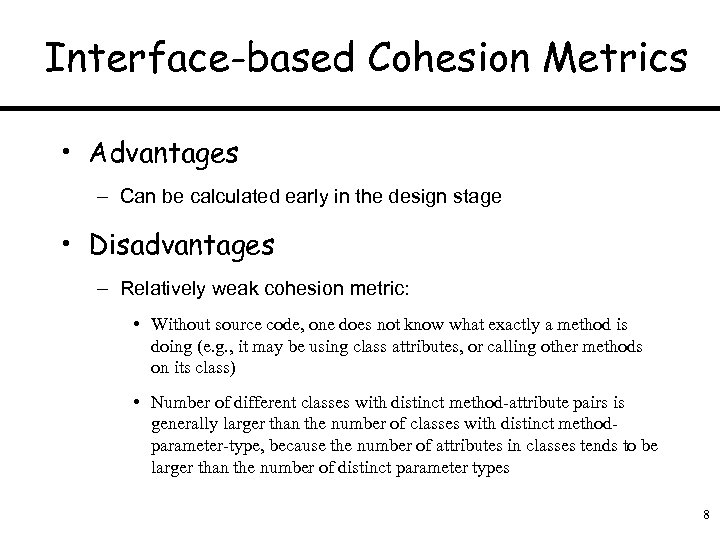Interface-based Cohesion Metrics • Advantages – Can be calculated early in the design stage • Disadvantages – Relatively weak cohesion metric: • Without source code, one does not know what exactly a method is doing (e. g. , it may be using class attributes, or calling other methods on its class) • Number of different classes with distinct method-attribute pairs is generally larger than the number of classes with distinct methodparameter-type, because the number of attributes in classes tends to be larger than the number of distinct parameter types 8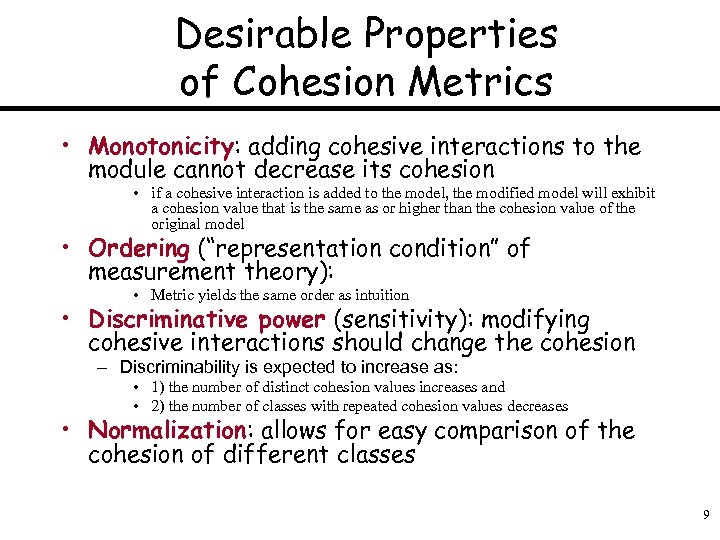Desirable Properties of Cohesion Metrics • Monotonicity: adding cohesive interactions to the module cannot decrease its cohesion • if a cohesive interaction is added to the model, the modified model will exhibit a cohesion value that is the same as or higher than the cohesion value of the original model • Ordering (“representation condition” of measurement theory): • Metric yields the same order as intuition • Discriminative power (sensitivity): modifying cohesive interactions should change the cohesion – Discriminability is expected to increase as: • 1) the number of distinct cohesion values increases and • 2) the number of classes with repeated cohesion values decreases • Normalization: allows for easy comparison of the cohesion of different classes 9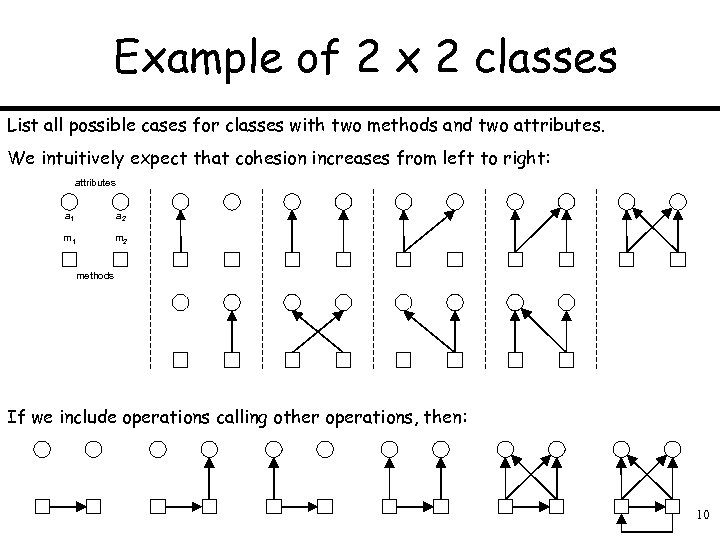Example of 2 x 2 classes List all possible cases for classes with two methods and two attributes. We intuitively expect that cohesion increases from left to right: attributes a 1 a 2 m 1 m 2 methods If we include operations calling other operations, then: 10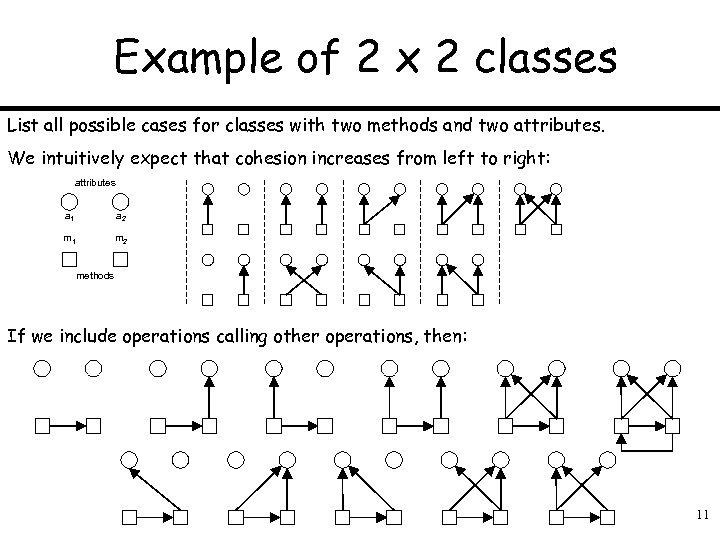Example of 2 x 2 classes List all possible cases for classes with two methods and two attributes. We intuitively expect that cohesion increases from left to right: attributes a 1 a 2 m 1 m 2 methods If we include operations calling other operations, then: 11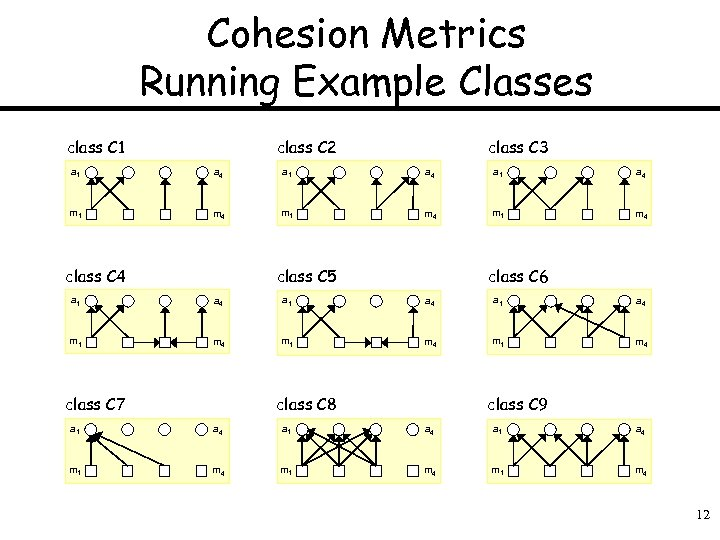Cohesion Metrics Running Example Classes class C 1 class C 2 class C 3 a 1 a 4 m 1 m 4 class C 5 class C 6 a 1 a 4 m 1 m 4 class C 7 class C 8 class C 9 a 1 a 4 m 1 m 4 12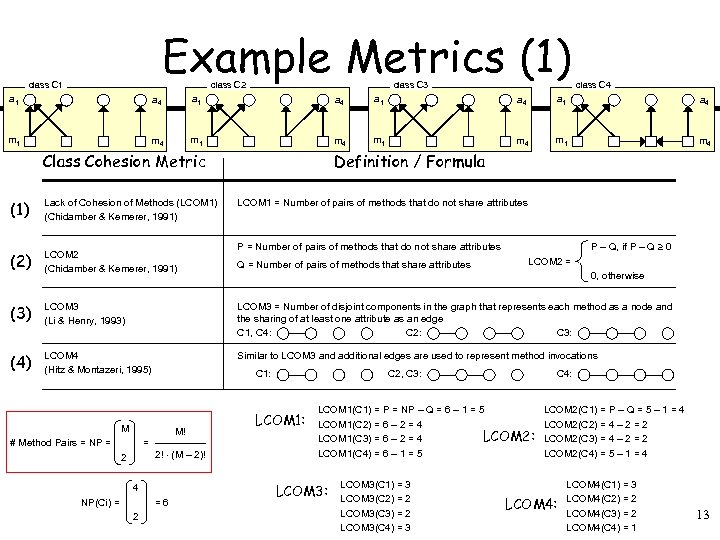Example Metrics (1) class C 1 class C 2 class C 3 class C 4 a 1 a 4 m 1 m 4 Class Cohesion Metric Definition / Formula (1) Lack of Cohesion of Methods (LCOM 1) (Chidamber & Kemerer, 1991) (2) LCOM 2 (Chidamber & Kemerer, 1991) (3) LCOM 3 (Li & Henry, 1993) LCOM 3 = Number of disjoint components in the graph that represents each method as a node and the sharing of at least one attribute as an edge C 1, C 4: C 2: C 3: (4) LCOM 4 (Hitz & Montazeri, 1995) Similar to LCOM 3 and additional edges are used to represent method invocations M! = 2 2! (M – 2)! 4 NP(Ci) = =6 2 P – Q, if P – Q 0 P = Number of pairs of methods that do not share attributes Q = Number of pairs of methods that share attributes C 1: M # Method Pairs = NP = LCOM 1 = Number of pairs of methods that do not share attributes LCOM 2 = 0, otherwise C 4: C 2, C 3: LCOM 1(C 1) = P = NP – Q = 6 – 1 = 5 LCOM 1(C 2) = 6 – 2 = 4 LCOM 2: LCOM 1(C 3) = 6 – 2 = 4 LCOM 1(C 4) = 6 – 1 = 5 LCOM 3: LCOM 3(C 1) = 3 LCOM 3(C 2) = 2 LCOM 3(C 3) = 2 LCOM 3(C 4) = 3 LCOM 2(C 1) = P – Q = 5 – 1 = 4 LCOM 2(C 2) = 4 – 2 = 2 LCOM 2(C 3) = 4 – 2 = 2 LCOM 2(C 4) = 5 – 1 = 4 LCOM 4: LCOM 4(C 1) = 3 LCOM 4(C 2) = 2 LCOM 4(C 3) = 2 LCOM 4(C 4) = 1 13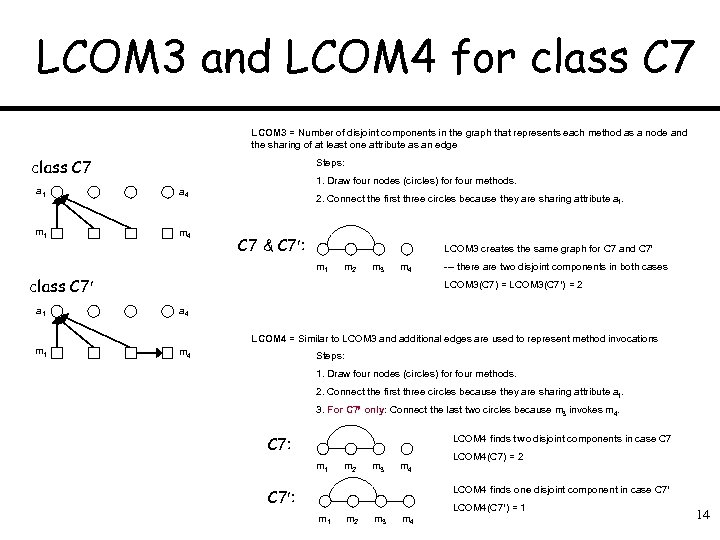LCOM 3 and LCOM 4 for class C 7 LCOM 3 = Number of disjoint components in the graph that represents each method as a node and the sharing of at least one attribute as an edge class C 7 Steps: a 1 m 4 1. Draw four nodes (circles) for four methods. a 4 2. Connect the first three circles because they are sharing attribute a 1. C 7 & C 7 : LCOM 3 creates the same graph for C 7 and C 7 m 1 m 2 m 3 m 4 class C 7 --- there are two disjoint components in both cases LCOM 3(C 7) = LCOM 3(C 7 ) = 2 a 1 a 4 m 1 m 4 LCOM 4 = Similar to LCOM 3 and additional edges are used to represent method invocations Steps: 1. Draw four nodes (circles) for four methods. 2. Connect the first three circles because they are sharing attribute a 1. 3. For C 7 only: Connect the last two circles because m 3 invokes m 4. C 7: LCOM 4 finds two disjoint components in case C 7 m 1 m 2 m 3 m 4 LCOM 4(C 7) = 2 LCOM 4 finds one disjoint component in case C 7 : m 1 m 2 m 3 m 4 LCOM 4(C 7 ) = 1 14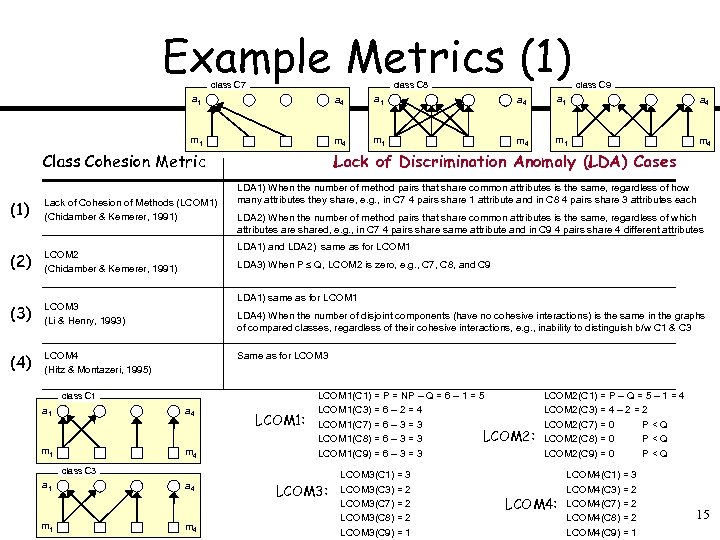Example Metrics (1) class C 7 class C 8 class C 9 a 1 a 4 m 1 m 4 Class Cohesion Metric (1) Lack of Cohesion of Methods (LCOM 1) (Chidamber & Kemerer, 1991) (2) LCOM 2 (Chidamber & Kemerer, 1991) (3) LCOM 3 (Li & Henry, 1993) (4) LCOM 4 (Hitz & Montazeri, 1995) Lack of Discrimination Anomaly (LDA) Cases LDA 1) When the number of method pairs that share common attributes is the same, regardless of how many attributes they share, e. g. , in C 7 4 pairs share 1 attribute and in C 8 4 pairs share 3 attributes each LDA 2) When the number of method pairs that share common attributes is the same, regardless of which attributes are shared, e. g. , in C 7 4 pairs share same attribute and in C 9 4 pairs share 4 different attributes LDA 1) and LDA 2) same as for LCOM 1 LDA 3) When P Q, LCOM 2 is zero, e. g. , C 7, C 8, and C 9 LDA 1) same as for LCOM 1 LDA 4) When the number of disjoint components (have no cohesive interactions) is the same in the graphs of compared classes, regardless of their cohesive interactions, e. g. , inability to distinguish b/w C 1 & C 3 Same as for LCOM 3 class C 1 a 4 m 1 m 4 LCOM 1: LCOM 1(C 1) = P = NP – Q = 6 – 1 = 5 LCOM 1(C 3) = 6 – 2 = 4 LCOM 1(C 7) = 6 – 3 = 3 LCOM 2: LCOM 1(C 8) = 6 – 3 = 3 LCOM 1(C 9) = 6 – 3 = 3 class C 3 a 1 m 1 a 4 m 4 LCOM 3: LCOM 3(C 1) = 3 LCOM 3(C 3) = 2 LCOM 3(C 7) = 2 LCOM 3(C 8) = 2 LCOM 3(C 9) = 1 LCOM 2(C 1) = P – Q = 5 – 1 = 4 LCOM 2(C 3) = 4 – 2 = 2 LCOM 2(C 7) = 0 P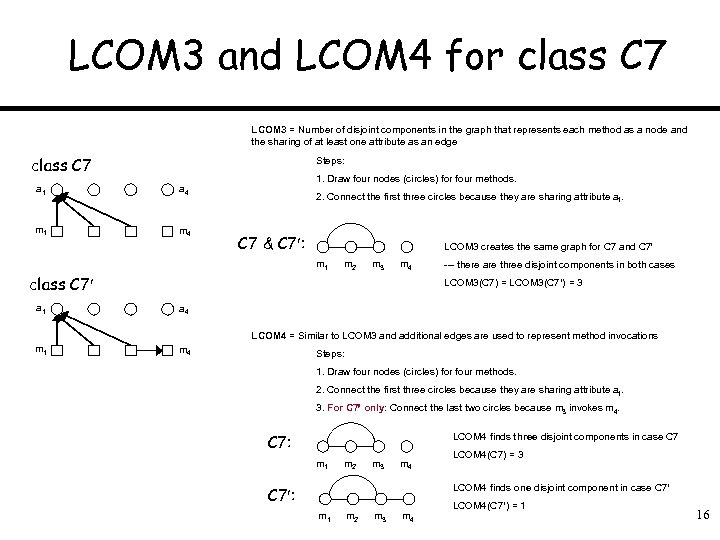LCOM 3 and LCOM 4 for class C 7 LCOM 3 = Number of disjoint components in the graph that represents each method as a node and the sharing of at least one attribute as an edge class C 7 Steps: a 1 m 4 1. Draw four nodes (circles) for four methods. a 4 2. Connect the first three circles because they are sharing attribute a 1. C 7 & C 7 : LCOM 3 creates the same graph for C 7 and C 7 m 1 m 2 m 3 m 4 class C 7 a 1 --- there are three disjoint components in both cases LCOM 3(C 7) = LCOM 3(C 7 ) = 3 a 4 LCOM 4 = Similar to LCOM 3 and additional edges are used to represent method invocations m 1 m 4 Steps: 1. Draw four nodes (circles) for four methods. 2. Connect the first three circles because they are sharing attribute a 1. 3. For C 7 only: Connect the last two circles because m 3 invokes m 4. C 7: LCOM 4 finds three disjoint components in case C 7 m 1 m 2 m 3 m 4 LCOM 4(C 7) = 3 LCOM 4 finds one disjoint component in case C 7 : m 1 m 2 m 3 m 4 LCOM 4(C 7 ) = 1 16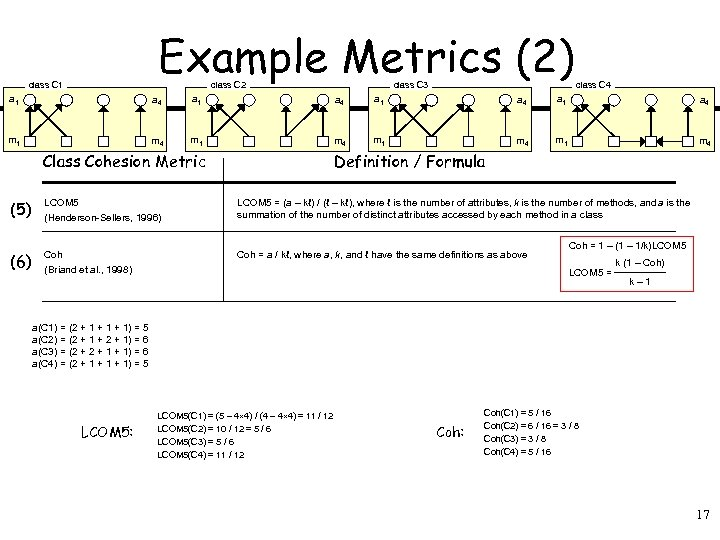Example Metrics (2) class C 1 class C 2 class C 3 class C 4 a 1 a 4 m 1 m 4 Class Cohesion Metric Definition / Formula (5) LCOM 5 (Henderson-Sellers, 1996) LCOM 5 = (a – kℓ) / (ℓ – kℓ), where ℓ is the number of attributes, k is the number of methods, and a is the summation of the number of distinct attributes accessed by each method in a class (6) Coh (Briand et al. , 1998) Coh = a / kℓ, where a, k, and ℓ have the same definitions as above Coh = 1 – (1 – 1/k)LCOM 5 = k (1 – Coh) k– 1 a(C 1) = (2 + 1 + 1) = 5 a(C 2) = (2 + 1 + 2 + 1) = 6 a(C 3) = (2 + 1 + 1) = 6 a(C 4) = (2 + 1 + 1) = 5 LCOM 5: LCOM 5(C 1) = (5 – 4 4) / (4 – 4 4) = 11 / 12 LCOM 5(C 2) = 10 / 12 = 5 / 6 LCOM 5(C 3) = 5 / 6 LCOM 5(C 4) = 11 / 12 Coh: Coh(C 1) = 5 / 16 Coh(C 2) = 6 / 16 = 3 / 8 Coh(C 3) = 3 / 8 Coh(C 4) = 5 / 16 17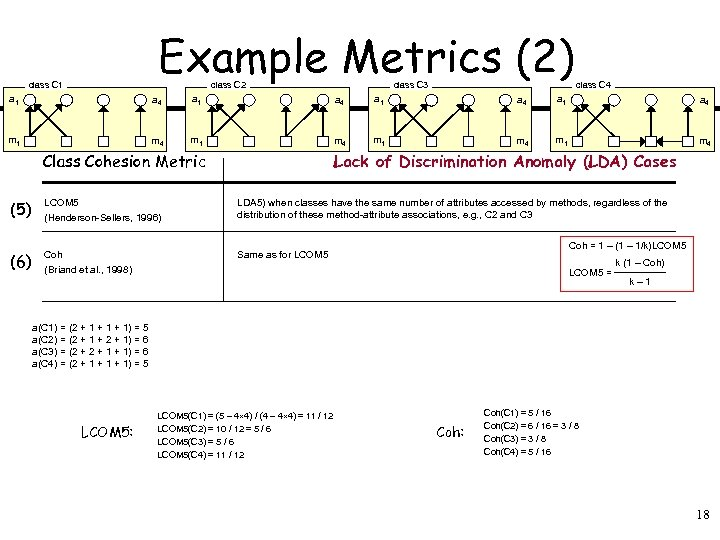Example Metrics (2) class C 1 class C 2 class C 3 class C 4 a 1 a 4 m 1 m 4 Class Cohesion Metric Lack of Discrimination Anomaly (LDA) Cases (5) LCOM 5 (Henderson-Sellers, 1996) LDA 5) when classes have the same number of attributes accessed by methods, regardless of the distribution of these method-attribute associations, e. g. , C 2 and C 3 (6) Coh (Briand et al. , 1998) Same as for LCOM 5 Coh = 1 – (1 – 1/k)LCOM 5 = k (1 – Coh) k– 1 a(C 1) = (2 + 1 + 1) = 5 a(C 2) = (2 + 1 + 2 + 1) = 6 a(C 3) = (2 + 1 + 1) = 6 a(C 4) = (2 + 1 + 1) = 5 LCOM 5: LCOM 5(C 1) = (5 – 4 4) / (4 – 4 4) = 11 / 12 LCOM 5(C 2) = 10 / 12 = 5 / 6 LCOM 5(C 3) = 5 / 6 LCOM 5(C 4) = 11 / 12 Coh: Coh(C 1) = 5 / 16 Coh(C 2) = 6 / 16 = 3 / 8 Coh(C 3) = 3 / 8 Coh(C 4) = 5 / 16 18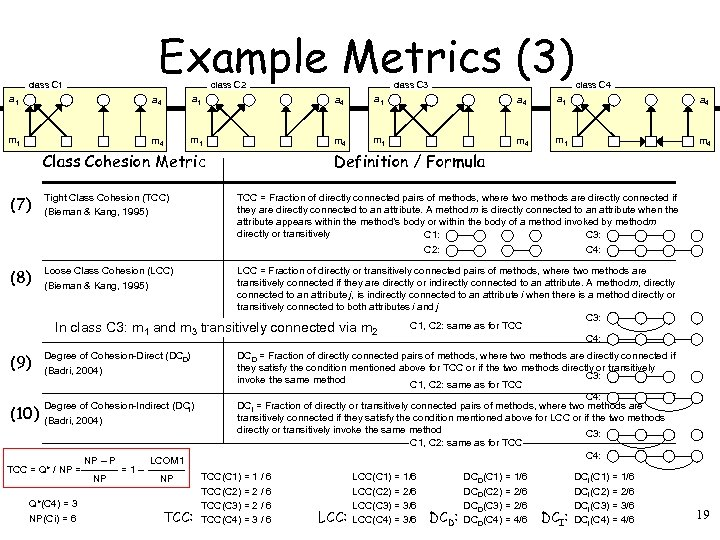Example Metrics (3) class C 1 class C 2 class C 3 class C 4 a 1 a 4 m 1 m 4 Class Cohesion Metric (7) Tight Class Cohesion (TCC) (Bieman & Kang, 1995) (8) Loose Class Cohesion (LCC) (Bieman & Kang, 1995) In class C 3: m 1 and TCC = Fraction of directly connected pairs of methods, where two methods are directly connected if they are directly connected to an attribute. A method m is directly connected to an attribute when the attribute appears within the method’s body or within the body of a method invoked by methodm directly or transitively C 1: C 3: C 4: C 2: LCC = Fraction of directly or transitively connected pairs of methods, where two methods are transitively connected if they are directly or indirectly connected to an attribute. A method m, directly connected to an attribute j, is indirectly connected to an attribute i when there is a method directly or transitively connected to both attributes i and j C 3: C 1, C 2: same as for TCC m 3 transitively connected via m 2 C 4: (9) Degree of Cohesion-Direct (DCD) (Badri, 2004) (10) Degree of Cohesion-Indirect (DCI) (Badri, 2004) TCC = Q* / NP = Q*(C 4) = 3 NP(Ci) = 6 NP – P NP =1– Definition / Formula LCOM 1 NP TCC: DCD = Fraction of directly connected pairs of methods, where two methods are directly connected if they satisfy the condition mentioned above for TCC or if the two methods directly or transitively C 3: invoke the same method C 1, C 2: same as for TCC C 4: DCI = Fraction of directly or transitively connected pairs of methods, where two methods are transitively connected if they satisfy the condition mentioned above for LCC or if the two methods directly or transitively invoke the same method C 3: C 1, C 2: same as for TCC C 4: TCC(C 1) = 1 / 6 TCC(C 2) = 2 / 6 TCC(C 3) = 2 / 6 TCC(C 4) = 3 / 6 LCC: LCC(C 1) = 1/6 LCC(C 2) = 2/6 LCC(C 3) = 3/6 LCC(C 4) = 3/6 DCD(C 1) = 1/6 DCD(C 2) = 2/6 DCD(C 3) = 2/6 DCD: DCD(C 4) = 4/6 DCI(C 1) = 1/6 DCI(C 2) = 2/6 DCI(C 3) = 3/6 DCI: DCI(C 4) = 4/6 19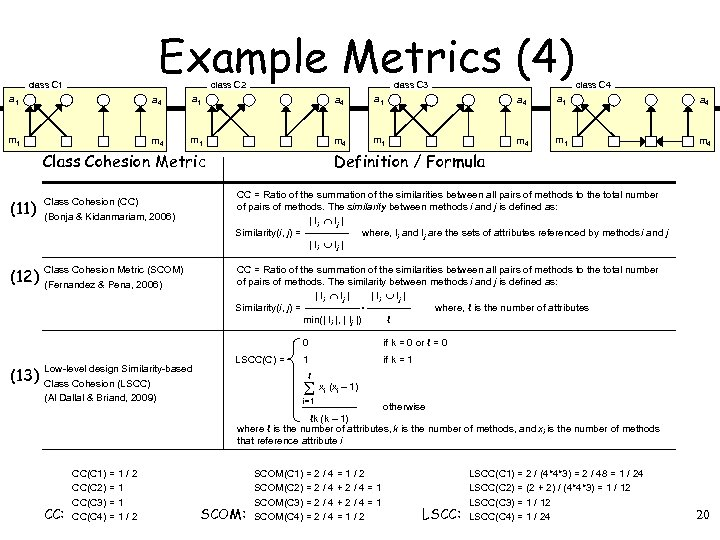Example Metrics (4) class C 1 class C 2 class C 3 class C 4 a 1 a 4 m 1 m 4 Class Cohesion Metric (11) Class Cohesion (CC) (Bonja & Kidanmariam, 2006) (12) Class Cohesion Metric (SCOM) (Fernandez & Pena, 2006) Definition / Formula CC = Ratio of the summation of the similarities between all pairs of methods to the total number of pairs of methods. The similarity between methods i and j is defined as: | Ii Ij | Similarity(i, j) = where, Ii and Ij are the sets of attributes referenced by methods i and j | Ii Ij | CC = Ratio of the summation of the similarities between all pairs of methods to the total number of pairs of methods. The similarity between methods i and j is defined as: | Ii Ij |. Similarity(i, j) = where, ℓ is the number of attributes min(| Ii |, | Ij |) ℓ 0 (13) Low-level design Similarity-based Class Cohesion (LSCC) (Al Dallal & Briand, 2009) CC: CC(C 1) = 1 / 2 CC(C 2) = 1 CC(C 3) = 1 CC(C 4) = 1 / 2 LSCC(C) = if k = 0 or ℓ = 0 1 if k = 1 ℓ x (x – 1) i i i=1 otherwise ℓk (k – 1) where ℓ is the number of attributes, k is the number of methods, and xi is the number of methods that reference attribute i SCOM: SCOM(C 1) = 2 / 4 = 1 / 2 SCOM(C 2) = 2 / 4 + 2 / 4 = 1 SCOM(C 3) = 2 / 4 + 2 / 4 = 1 SCOM(C 4) = 2 / 4 = 1 / 2 LSCC: LSCC(C 1) = 2 / (4*4*3) = 2 / 48 = 1 / 24 LSCC(C 2) = (2 + 2) / (4*4*3) = 1 / 12 LSCC(C 4) = 1 / 24 20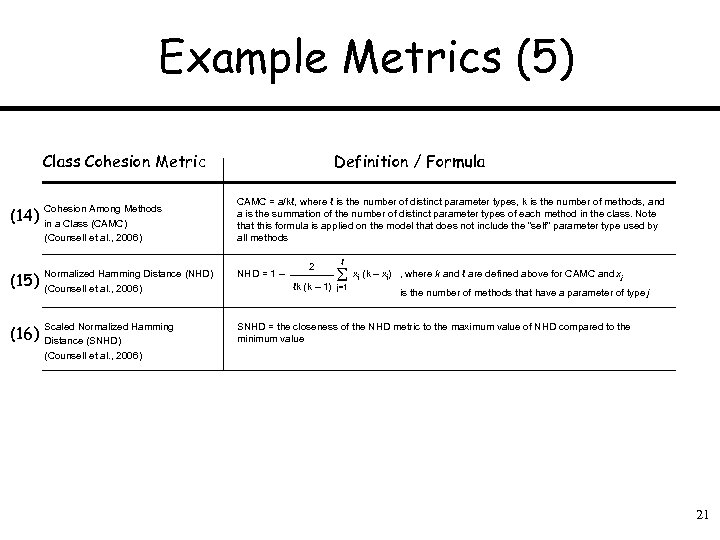Example Metrics (5) Class Cohesion Metric Definition / Formula CAMC = a/kℓ, where ℓ is the number of distinct parameter types, k is the number of methods, and a is the summation of the number of distinct parameter types of each method in the class. Note that this formula is applied on the model that does not include the “self” parameter type used by all methods (14) Cohesion Among Methods in a Class (CAMC) (Counsell et al. , 2006) (15) Normalized Hamming Distance (NHD) (Counsell et al. , 2006) NHD = 1 – (16) Scaled Normalized Hamming Distance (SNHD) (Counsell et al. , 2006) SNHD = the closeness of the NHD metric to the maximum value of NHD compared to the minimum value 2 ℓ x (k – x ) j ℓk (k – 1) j=1 j , where k and ℓ are defined above for CAMC and xj is the number of methods that have a parameter of type j 21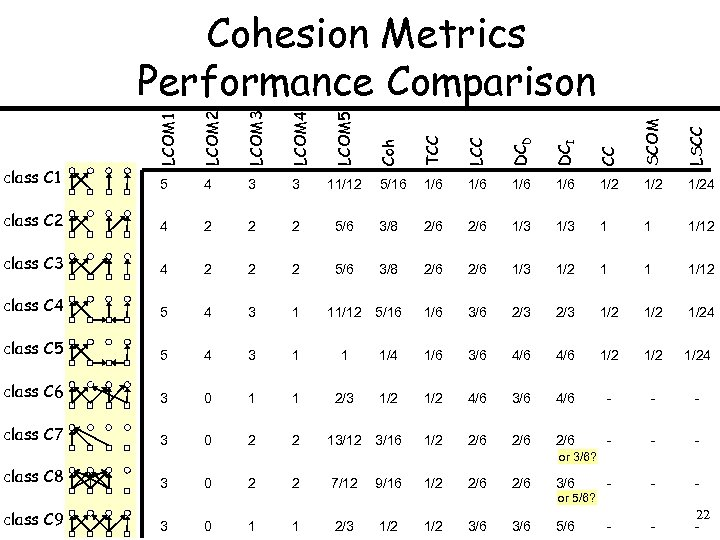2 2 2 class C 3 4 2 2 2 class C 4 5 4 3 1 class C 5 5 4 3 1 1 class C 6 3 0 1 1 2/3 class C 7 3 0 2 2 LSCC 4 SCOM class C 2 CC 11/12 DCI LCOM 5 3 DCD LCOM 4 3 LCC LCOM 3 4 TCC LCOM 2 5 class C 1 Coh LCOM 1 Cohesion Metrics Performance Comparison 5/16 1/6 1/6 1/2 1/24 5/6 3/8 2/6 1/3 1 1 1/12 5/6 3/8 2/6 1/3 1/2 1 1 1/12 11/12 5/16 1/6 3/6 2/3 1/2 1/24 1/6 3/6 4/6 1/2 1/24 1/2 4/6 3/6 4/6 - - - 13/12 3/16 1/2 2/6 2/6 - - - or 3/6? class C 8 class C 9 3 0 2 2 7/12 9/16 1/2 2/6 3/6 - - - 3 0 1 1 2/3 1/2 3/6 5/6 - - 22 - or 5/6?